Next: FORTRAN source file Up: Solution of the Skyrme-Hartree-Fock-Bogolyubov Previous: Starting the iteration

# Output file

Together with the FORTRAN source code in the file hfodd.f, an example of the output file is provided in la132-a.out. Selected lines from this file are presented in section TEST RUN OUTPUT below. This output file corresponds to the input file la132-a.dat reproduced in section TEST RUN INPUT below.

The output file contains two consecutive runs of the code HFODD. The first run starts from the Nilsson model, see Sec. I-3.10, and in 95 iterations finds a triaxial solution in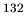La for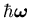=0. The triaxial shape is forced by using appropriate constraints for moments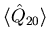and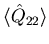. Such a solution is obtained with parity and signature symmetries conserved, and with the time-reversal broken in order to describe odd numbers of neutrons and protons. Therefore, even for=0 one obtains solution with a non-zero value of the angular momentum, which is oriented along the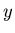axis. Since this first run is performed within conditions that have already been available in the previous version (v1.75r), in section TEST RUN OUTPUT we skip the corresponding output lines.

The second run starts from the solution obtained in the first run and performs calculations with all symmetries broken except parity, and for the initial angular momentum vector of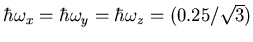MeV. Since for such a low value of the angular frequency, only a planar solution exists , the nucleus has to turn in space in such way that the angular momentum vector coincides with the symmetry plane spanned by the short and long axes of the mass distribution. Standard iteration, with IMOVAX=0, see Secs. 2.3 and 3.5, requires several thousand iterations to achieve the alignment of the angular momentum and angular frequency vectors below the angle of 0.01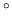. On the other hand, iteration with IMOVAX=1, as shown in the example of file la132-a.dat, achieves the alignment of 0.000001within 92 iterations.

Section SKYRME FORCE DEFINITION lists the name and parameters of the Skyrme force together with parameters KETA_J, KETA_W, KETACM, and KETA_M that define the way the given force should be used (see Sec. 3.1).

Section CALCULATIONS WITH THE TILTED-AXIS CRANKING gives values of components of the angular frequency vector and its length. For switches IMOVAX=1 or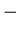1 and IOCONT=0, these vales are used only in the first iteration, and later ignored, because the angular frequency vector is in each iteration readjusted to be aligned or anti-aligned with the angular momentum vector.

Section PARITY CONFIGURATIONS gives numbers of neutron and proton states in the two parity blocks, see Sec. 3.3.

Section CONVERGENCE REPORT gives information on the performed iterations. One line per one iteration lists values described in Sec. II-4, except that version (v2.07f) prints the multipole moments in the intrinsic frame, the last before last column gives the angle between the angular frequency and angular momentum vectors, and the last column gives the total pairing energy.

Section SINGLE-PARTICLE PROPERTIES gives information on the s.p. states. For broken simplex symmetry, three projections of the total angular momentum and intrinsic spin are printed in the first and second line, respectively, for each s.p. state.

Section EULER ANGLES OF THE PRINCIPAL-AXES FRAME gives the standard Euler angles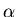,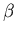, and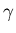in degrees, see Sec. 2.4, which define the orientation of the principal-axes (intrinsic) frame of reference with respect to the original frame.

Section MULTIPOLE MOMENTS [UNITS: (10 FERMI)^ LAMBDA] gives values of multipole moments with respect to the original frame of reference. Similarly, section MULTIPOLE MOMENTS [UNITS: (10 FERMI)^ LAMBDA] [INTRINSIC FRAME] gives analogous values with respect to the intrinsic frame of reference. Whenever the parity symmetry is broken, yet another analogous section gives information on values of multipole moments with respect to the center-of-mass reference frame.

Sections MAGNETIC MOMENTS [MAGNETON*FERMI^ (LAMBDA-1)]  and   MAGNETIC MOMENTS [MAGNETON*FERMI^ (LAMBDA-1)] [INTRINSIC FRAME] give values of magnetic moments with respect to the original and intrinsic reference frame, respectively.

Section ANGULAR MOMENTA IN THE THREE CARTESIAN DIRECTIONS gives values of neutron, proton, and total projections of the total angular momentum and intrinsic spin on the three Cartesian axes.

Sections NEUTRON CONFIGURATIONS and PROTON CONFIGURATIONS give visual representation of states occupied in the parity blocks, in analogy to those described in Sec. I-4.

Section ENERGIES gives summary of energies calculated in the last iteration. Apart from entries described in Sec. I-4, it gives in addition the pairing rearrangement energies P-REARR, pairing gaps PAIRGAP, Fermi energies E-FERMI, and surface multipole constraint energies CONSTR. (SURF).

Alternatively, one can perform the second run with the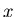-simplex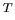conserved (ISIMTX=1) and the initial angular frequency vector of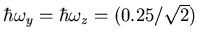MeV and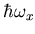=0. In this way, the angular momentum is confined within the symmetry plane-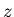spanned by the short and long axes of the mass distribution. The corresponding input and output data files are provided in files la132-b.dat and la132-b.out, respectively. Since the planar solution in question corresponds to projections of the angular momentum on the short and long axes that are not equal to one another, the nucleus has now turn in space about theaxis. For IMOVAX=0, this process is also very slow, while for IMOVAX=1, as in file la132-b.dat it takes again only 92 iterations. Apart from different orientations in space, solutions obtained by using input data files la132-a.dat and la132-b.dat are entirely equivalent. Output file la132-b.out is not shown in section TEST RUN OUTPUT below.Next: FORTRAN source file Up: Solution of the Skyrme-Hartree-Fock-Bogolyubov Previous: Starting the iteration
Jacek Dobaczewski 2004-01-06